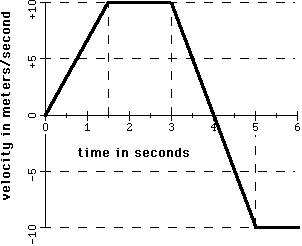# Accerlation Question

An automobile moves forward and backward on a straight highway.The graph shows the velocity of this automobile as a function of time.What is the acceleration of the automobile at t = 4 s? (Round to two significant digits.)

a = change in velocity / time

I said the acceleration was zero, because the automobile would be stopped at t = 4 s. This is wrong, however.

Dick
Homework Helper
An automobile moves forward and backward on a straight highway.The graph shows the velocity of this automobile as a function of time.What is the acceleration of the automobile at t = 4 s? (Round to two significant digits.)

a = change in velocity / time

I said the acceleration was zero, because the automobile would be stopped at t = 4 s. This is wrong, however.

Of course it is wrong. The automobile may be stopped, but acceleration is the rate of change of velocity. It's just in between moving forwards and moving backwards. Try that again.

Of course it is wrong. The automobile may be stopped, but acceleration is the rate of change of velocity. It's just in between moving forwards and moving backwards. Try that again.

The question is asking for the acceleration at exactly 4 s, so if I used the formula a = v/t I would get a = 0/4, which is 0.

The answer is -10, but I don't understand why when that acceleration is reached a second later.

Dick